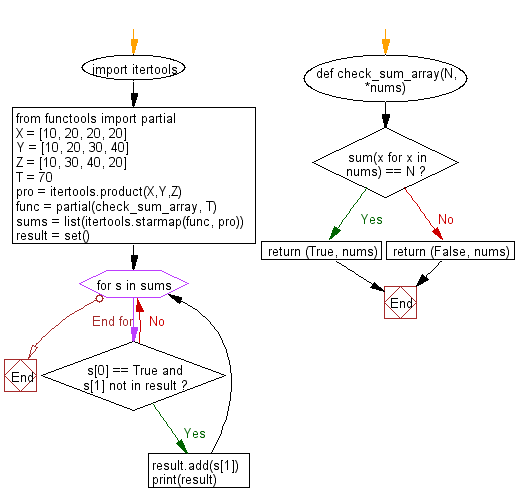﻿ Python: Check the sum of three elements from three arrays is equal to a target value - w3resource# Python: Check the sum of three elements from three arrays is equal to a target value

## Python Basic - 1: Exercise-11 with Solution

Write a Python program to check the sum of three elements (each from an array) from three arrays is equal to a target value. Print all those three-element combinations.

Sample data:
/*
X = [10, 20, 20, 20]
Y = [10, 20, 30, 40]
Z = [10, 30, 40, 20]
target = 70
*/

Sample Solution:

Python Code:

``````import itertools
from functools import partial
X = [10, 20, 20, 20]
Y = [10, 20, 30, 40]
Z = [10, 30, 40, 20]
T = 70

def check_sum_array(N, *nums):
if sum(x for x in nums) == N:
return (True, nums)
else:
return (False, nums)
pro = itertools.product(X,Y,Z)
func = partial(check_sum_array, T)
sums = list(itertools.starmap(func, pro))

result = set()
for s in sums:
if s == True and s not in result:
print(result)
``````

Sample Output:

```{(10, 20, 40)}
{(10, 20, 40), (10, 30, 30)}
{(10, 20, 40), (10, 30, 30), (10, 40, 20)}
{(10, 20, 40), (10, 30, 30), (20, 10, 40), (10, 40, 20)}
{(10, 20, 40), (20, 20, 30), (10, 30, 30), (20, 10, 40), (10, 40, 20)}
{(10, 20, 40), (10, 40, 20), (20, 10, 40), (10, 30, 30), (20, 20, 30), (20, 30, 20)}
{(10, 20, 40), (10, 40, 20), (20, 10, 40), (20, 40, 10), (10, 30, 30), (20, 20, 30), (20, 30, 20)}
```

Flowchart:## Visualize Python code execution:

The following tool visualize what the computer is doing step-by-step as it executes the said program:

Python Code Editor :

Have another way to solve this solution? Contribute your code (and comments) through Disqus.

What is the difficulty level of this exercise?

Test your Programming skills with w3resource's quiz.

﻿

## Python: Tips of the Day

Iterating over dictionaries using 'for' loops:

I am a bit puzzled by the following code: d = {'x': 1, 'y': 2, 'z': 3} for key in d: print key, 'corresponds to', d[key] What I don't understand is the key portion. How does Python recognize ...

key is just a variable name.

```for key in d:
```

For Python 3.x:

```for key, value in d.items():
```

For Python 2.x:

```for key, value in d.iteritems():
```

To test for yourself, change the word key to poop.

In Python 3.x, iteritems() was replaced with simply items(), which returns a set-like view backed by the dict, like iteritems() but even better. This is also available in 2.7 as viewitems().

The operation items() will work for both 2 and 3, but in 2 it will return a list of the dictionary's (key, value) pairs, which will not reflect changes to the dict that happen after the items() call. If you want the 2.x behavior in 3.x, you can call list(d.items()).

Ref: https://bit.ly/37dm0Qo